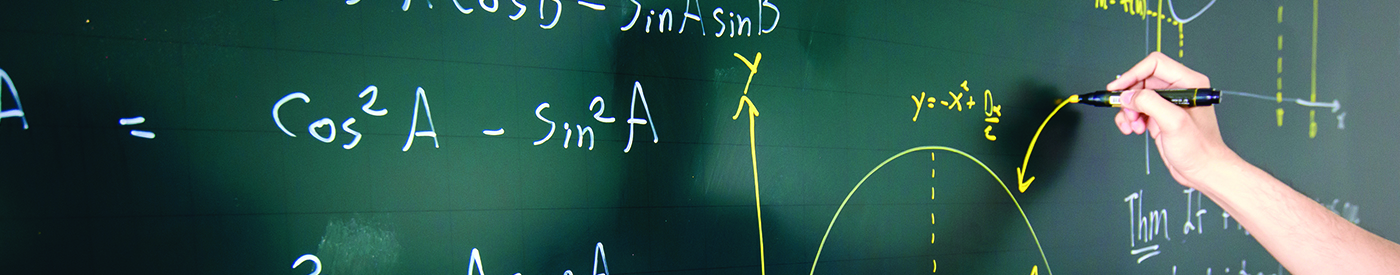## Fusion Math P3 (9 yr old)

##### Objectives
1. Develop a strong foundation in Mathematical topics related to Math competitions.
2. Expose students to various Mathematical concepts beyond school syllabus.
3. Equip students with essential skills needed to tackle challenging Mathematical problems.

All P3 students who are keen in Mathematics are encouraged to participate in this programme. Students younger than 9 years old are required to pass a Modular Diagnostic Paper (MDP). This is to ensure the younger students have the fundamental foundation to manage.

Year End Holiday Semester 1 (Jan – Mar)
– Multiply
– Divide
– Problem Solving on Whole Number
– Fractions
– Box Sums
– Summation
– Four Operations on Whole Numbers
– Four Operations on Fractions
– Four Operations on Decimals
– Problem Solving on Fractions (Addition / Subtraction)
– Problem Solving on Fractions (Models, Units)
– Assumption Method
– Factors & Multiples
March Holiday Semester 1 (Mar – May)
– Alphametics (Multiplication)
– Number Grouping
– Counting Triangles
– Counting Squares
– Counting Rectangles
– Calendar
– Grouping
– Balancing the Table
– Number of Terms
– Number Summation
Mid Year Holiday Semester 2 (Jun – Sep)
– Four Operations on Whole Number
– Four Operations on Fraction
– Four Operations on Decimal
– Next Number
– Calendar
– Area & Perimeter
– Average
– Counting Routes
– Box Sum (Multiplication & Division)
– Problem Solving
– Fraction & Ratio Concept
– Problem Solving on Time
– Rate
– Average (Middle Number)
September Holiday Semester 2 (Sep – Nov)
– Number of Terms
– Summation
– Counting Triangles
– Counting Squares
– Counting Rectangles
– Rate
– Angles
– Volume
– Surface Area
– Area & Perimeter on Triangles
– Conversion
– Problem Solving

Topics covered are subjected to change depending on the ability of students.